The Collatz conjecture

Posted on Sun 02 June 2019 in Math

Introduction

I came across the Collatz conjecture reading the book The Model Thinker. The book I don’t recommend particulary, 80% of it is topics science/engineering students learn at college (eg. entropy, normal distribution). But 20% is interesting tidbits and references I haven’t heard of. One was the the Collatz conjecture. From wikipedia:

The Collatz conjecture is a conjecture in mathematics that concerns a sequence defined as follows: start with any positive integer n. Then each term is obtained from the previous term as follows: if the previous term is even, the next term is one half the previous term. If the previous term is odd, the next term is 3 times the previous term plus 1. The conjecture is that no matter what value of n, the sequence will always reach 1.

The kicker is, nobody can prove the Collatz conjecture. Not even Terry Tao!

Here it is as code, so we can play around with it. The code is up on Github:

def collatz(n):
if n % 2 == 0: return int(n/2)
else:          return 3*n+1

def collatz_sequence(n):
sequence = [n]
while n != 1:
n = collatz(n)
sequence += [n]
return sequence

For example:

collatz_sequence(7)
[7, 22, 11, 34, 17, 52, 26, 13, 40, 20, 10, 5, 16, 8, 4, 2, 1]

How about a bigger one:

collatz_sequence(2498904803)
[2498904803,
7496714410,
3748357205,
11245071616,
5622535808,
2811267904,
1405633952,
702816976,
...
53,
160,
80,
40,
20,
10,
5,
16,
8,
4,
2,
1]

The one above is 278 long! This is what it looks like on a log plot:

plt.plot([log2(i) for i in collatz_sequence(2498904803)])Sequence properties

The sequences have a lot of random properties. For example, plotting the length of Collatz sequences:

plt.plot([len(collatz_sequence(i)) for i in range(1, 2**11)])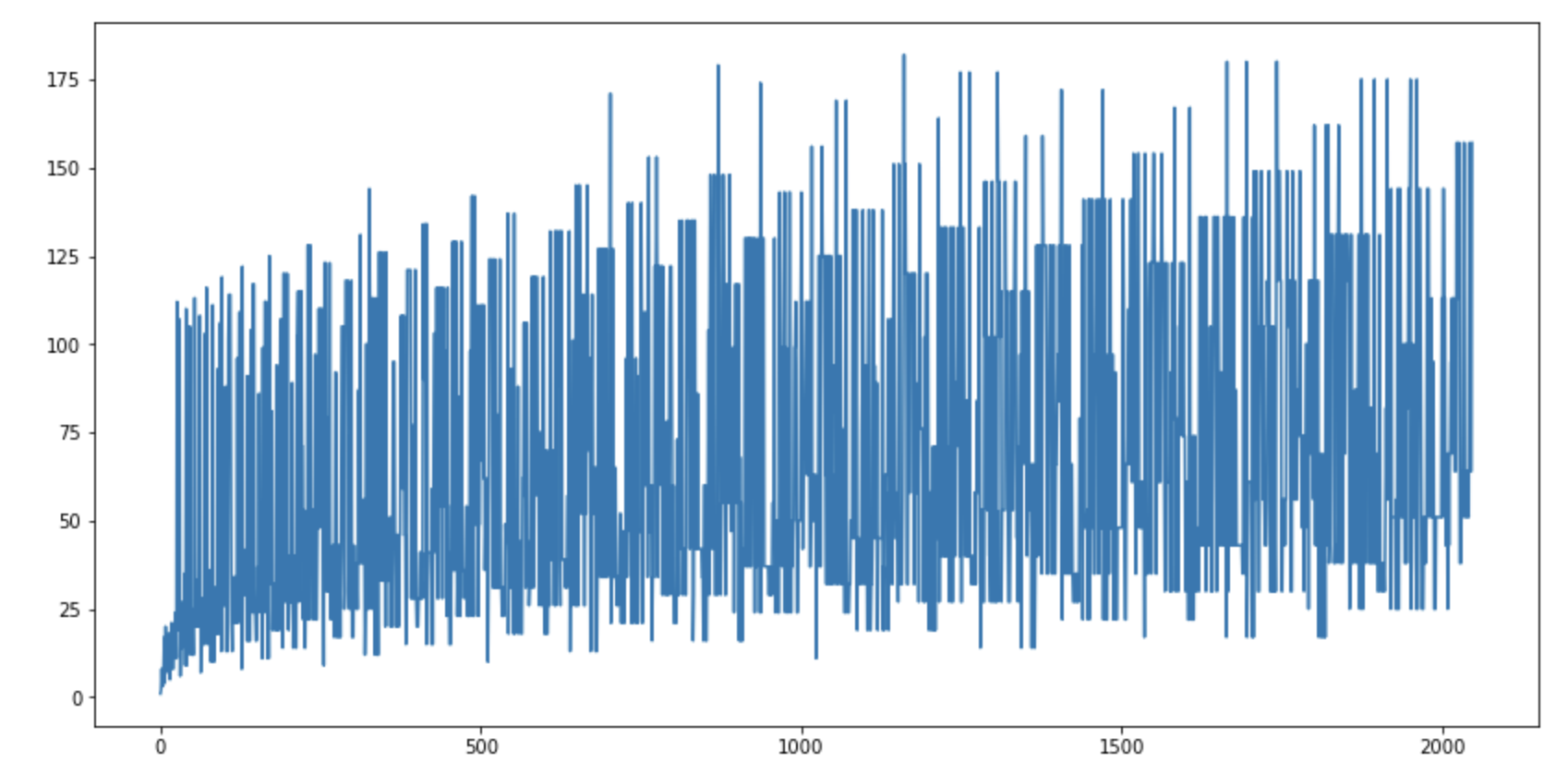What is the biggest value the sequence gets to, on a log plot:

plt.plot([log2(max(collatz_sequence(i))) for i in range(1, 2**11)])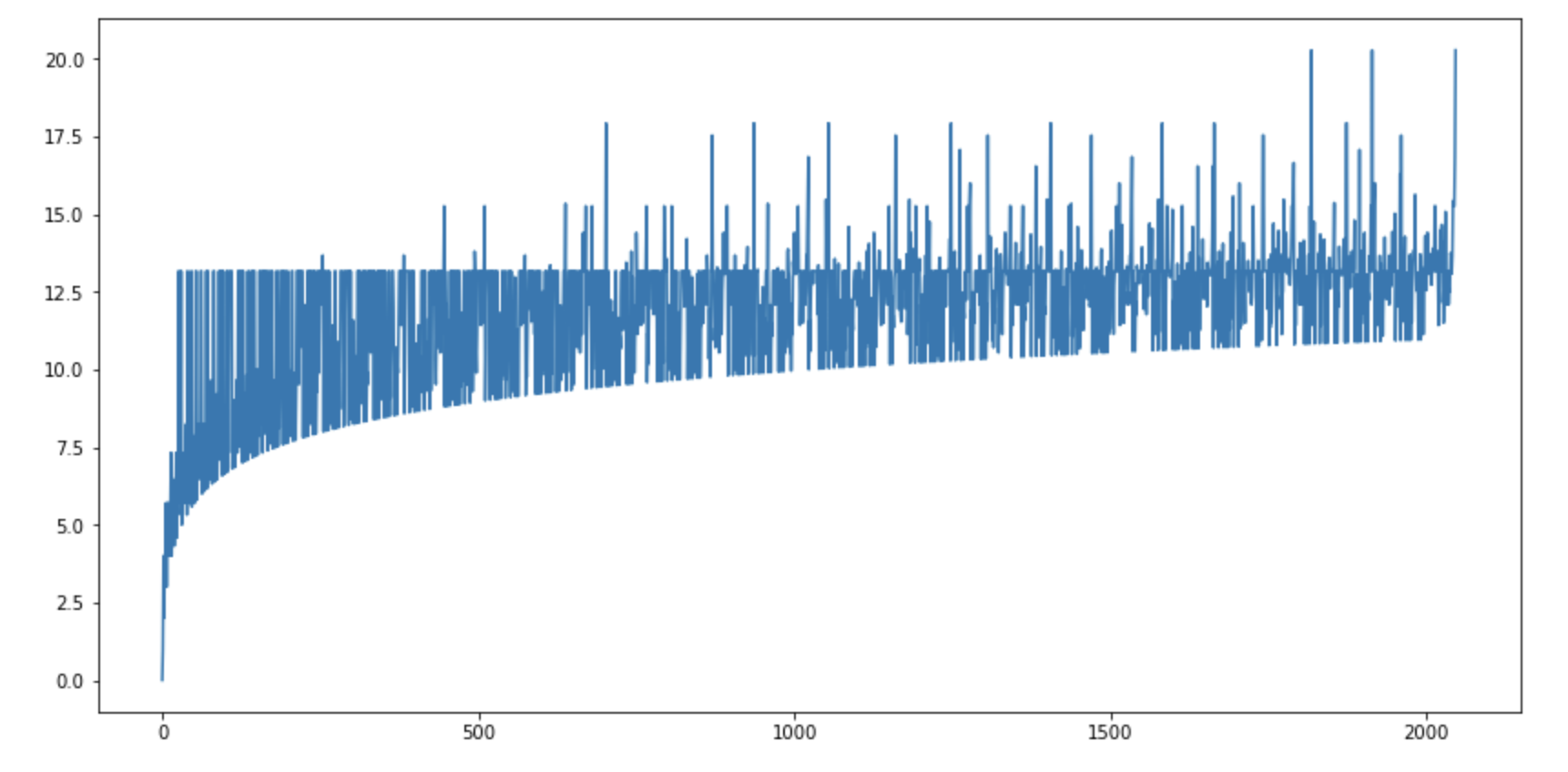Some people are brute-forcing it, and have checked that all numbers satisfy the conjecture up to about 2^64. They're also recording path records, ie. the biggest number reached on the path down to 1. The current path record is (this is the max() I plotted above), reading off from the linked page (without running):

max(collatz_sequence(71,149323,674102,624415)) == 9055,383924,226744,340579,466230,337749,396932

Plotting the path records on a log-log plot looks very linear: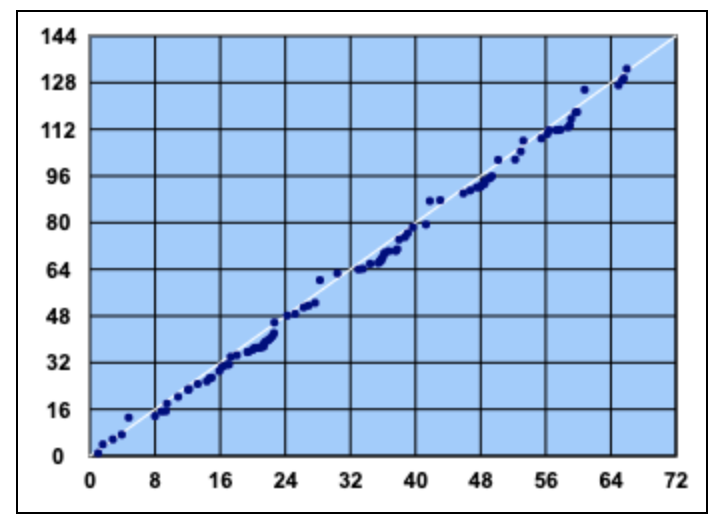The only way the series can get down to 1 is if it decreases. It only decreases when dividing by 2. So another way to state the conjecture is that eventually all series reach a number that is 2^k for some integer k. So let's check what is the biggest k, as a function of n:

plt.plot([log2(max_power2(collatz_sequence(i))) for i in range(1, 2**16)])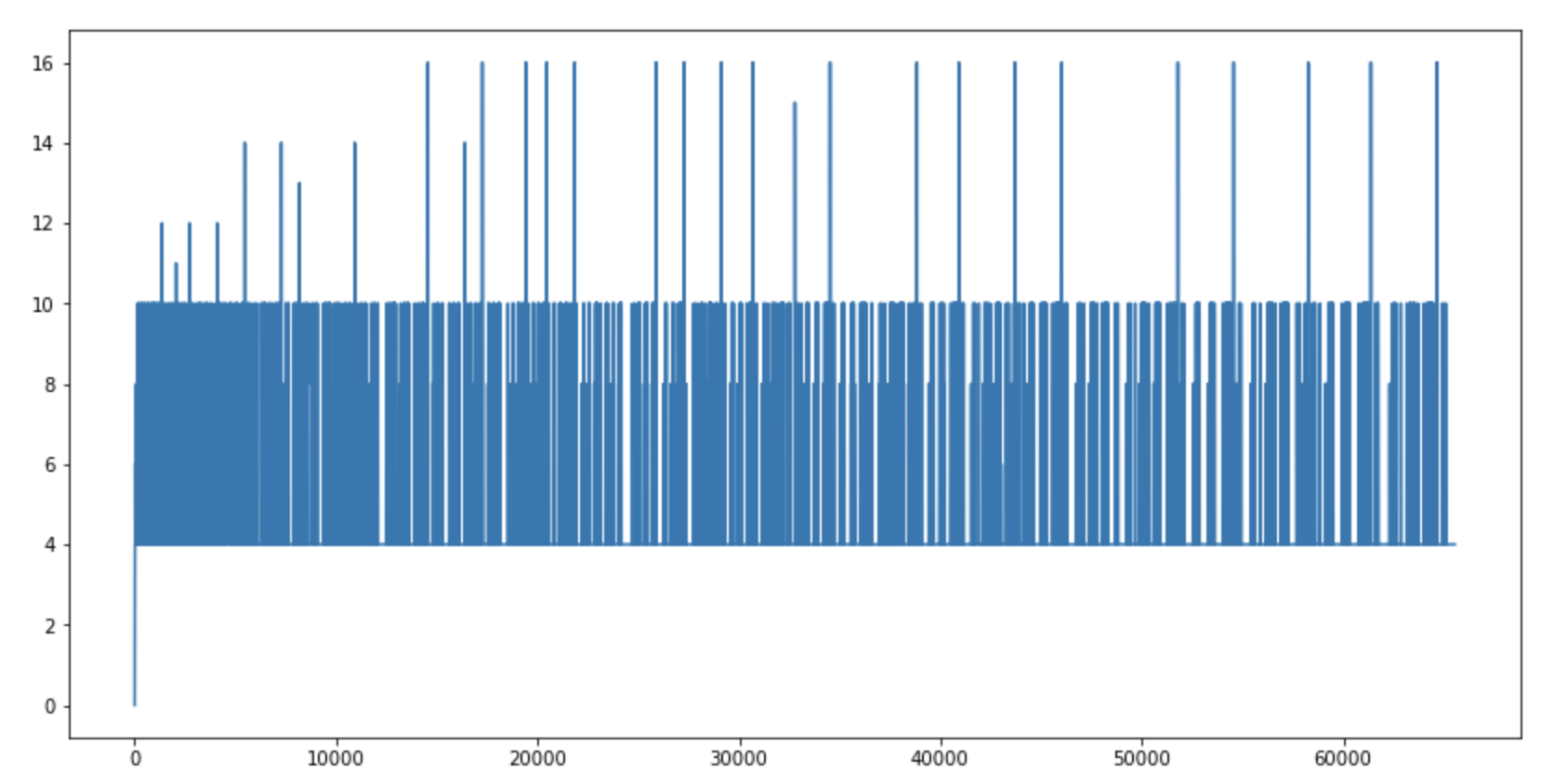It would be nice if there would be some regularity here, like numbers smaller than 2^k end up in 2^k, but that's not true. However, something similar was found by the brute-force check project:

The table therefore establishes the practical fact that for all numbers in the interval researched so far the path of every number taking k bytes (assuming a byte consists of 8 bits) can be completely determined using a storage of just 2k bytes for intermediate results.

Another idea I had was around Lyapunov functions. If one can come up with a function L(), so that L() is decreasing for a Collatz sequence (and L() has some good properties), that could help. One thing I tried is counting how the sequence produces 1s in the binary representation of the number:

plt.plot(
[
max(
[
'{0:b}'.format(k).count('1') for k in collatz_sequence(i)
]) - '{0:b}'.format(i).count('1') for i in range(1, 2**16)
])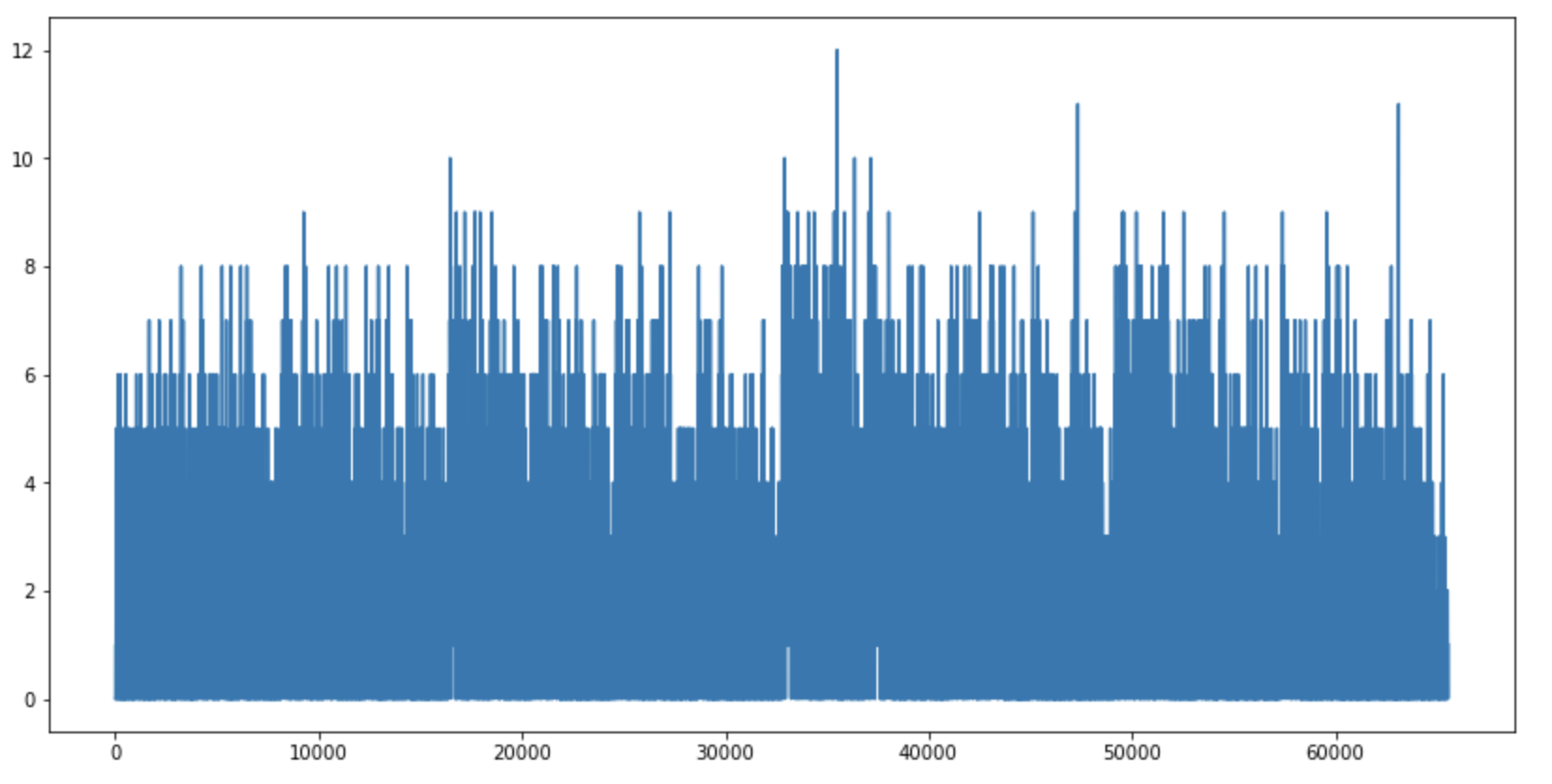The 1s are not conserved. It is possible to upper bound it, for example:

plt.plot(
[
len('{0:b}'.format(i)) + '{0:b}'.format(i).count('1') - max(
[
'{0:b}'.format(k).count('1') for k in collatz_sequence(i)
]) for i in range(1, 2**16)
])But this isn't actually useful (not a good Lyapunov function), since the binary 1s can keep traveling up in the binary sequence, so the numbers can keep getting bigger.

Primes and cycles

Another way to think about it like this: imagine the number broken out as prime factors. When there are prime factors like 2^k, those are chopped off by the divisions. So any time the sequence hits a number where the prime factors have a 2^k, those are chopped off. If there is something left (not 1), it will be odd, let's call it n=m+1, where m is even. We multiply it by 3 and add 1 (3m+4), that will be even, so we divide by 2. So what do we know about (3m+4)/2 = m + m/2 + 2, if m is even? I don't know, but other people are also thinking about this.

Taking one step back, if n is odd, it does not have 2 as a factor. We multiply it by 3, so we add 3 to the prime factors. Then we add 1, what happens to the prime factors? I don't know, nobody knows!

Another thing to keep in mind is cycles:

Any counterexample to the Collatz conjecture would have to consist either of an infinite divergent trajectory or a cycle different from the trivial (4; 2; 1) cycle. Thus, if one could prove that neither of these types of counterexample could exist, then all positive integers would have a trajectory that reaches the trivial cycle. Such a strong result is not known, but certain types of cycles have been ruled out.

To prove that it comes down to 1, we have to prove there are no cycles, if there's no number k that is reached (by 3n+1'ing a smaller number), which eventually will be reached again (by dividing 2k by 2), and then the sequence keeps looping. The only known cycle is the trivial: [1 → 4 → 2 → 1]. There's also no proof about no cycles.

Happy Collatz'ing! ☺